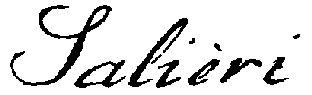[Contents] · [Home] · [Mail]

## SALIERI Language - integer (Object Type)

Objects of this type have integer values between -2147483648 (= -2^31) and +2147483647 (= 2^31-1). Integer constants may have an arbitrary number of positive (+) or negative (-) signs, which are treated as prefix operators and resolved according to the usual rules (i.g., +12 = 12; --345 = 345).

The basic operations on integers are addition (+), subtraction (-), multiplication (*), integer division (div), and division remainder (mod). The precedence of these operators is as usual (unary - and + binding strongest, then *, mod, and div, and finally binary + and - with the weakest precedence).

Attention!
When using integers, keep in mind, that their range is limited (see above); if by application of an operation (like + or *) this range is exceeded, no error condition occurs, but incorrect results are produced. If you have to use larger numbers, you should use objects of type real.

Example:
> +234
Result= 234
> -+-234
Result= 234
> 7 + 10 div 3 * 12 - 1
Result= 42

See also: +, -, *, mod, div, Operators and Expressions.

[Contents] · [Home] · [Mail]
© sic!systems, page frame designed by hh; this page has been automatically generated from the SALIERI Documentation Database.